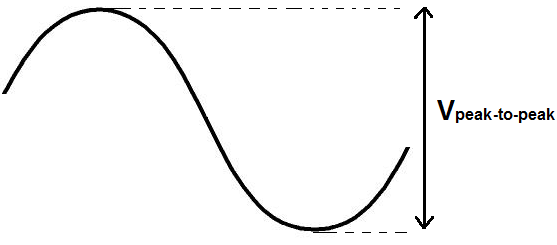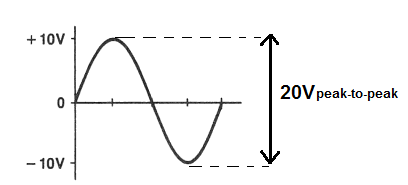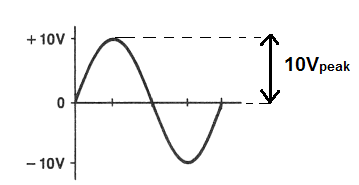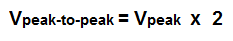﻿ What is Peak-to-Peak Voltage (Vpp)? ﻿# What is Peak-to-Peak Voltage (VPP)?Peak-to-peak voltage, VPP, is a voltage waveform which is measured from the top of the waveform, called the crest, all the way down to the bottom of the waveform, called the trough.

You can see that all this is shown in the above diagram.

So peak-to-peak voltage is just the full vertical length of a voltage waveform from the very top to the very bottom.

Below we show another sine waveform but this time with numerical values, to serve as a mathematical illustration of peak-to-peak voltage:You can see that the voltage waveform above reaches a top peak, or crest, of 10V of positive voltage and a bottom peak, or trough, of -10V. Therefore, this waveform above has a peak-to-peak waveform of 10V-(-10V)=20V. This VPP of 20V represents the entire vertical length of the voltage of this waveform.

There is another type of voltage called the peak voltage. Sometimes peak voltage and peak-to-peak voltage are confused with each other. We will show the difference between peak voltage and peak-to-peak voltage. While peak-to-peak voltage is the voltage from the trough of the waveform all the way to the crest, the peak voltage is the voltage from the 0 reference line to the positive peak of the voltage waveform. Therefore, the peak voltage is exactly half of the peak-to-peak voltage waveform. The waveform above is now shown below with regard to peak voltage, not peak-to-peak.So peak-to-peak voltage is exactly double the value of the peak voltage of a waveform.

### Examples of Peak-to-Peak Voltage

You will encounter peak-to-peak voltage in many environments. However, never assume a voltage is peak-to-peak unless directly specified as that. For example, the voltage should say at the end, VPP. For example, 60VPP.

Let's go over a real life example of VPP in an item you see regularly in buildings everywhere, including your home- an AC outlet. As you may know, an AC oulet outputs 120V. This voltage is not the peak-to-peak voltage. This is the RMS voltage. If you need an explanation of RMS voltage, see RMS Voltage and Current-Explained.. This same 120Vrms is 170V in peak voltage. So in peak-to-peak voltage, it is 340 volts. So the voltage which comes out of your AC outlet (United States) is 340Vpeak-to-peak. This is just a common example.

### How to Calculate Peak-to-Peak Voltage

Peak-to-peak voltage can be calculated either from the peak voltage, the rms (root mean square) voltage, or the average voltage.

The formulas to calculate the VPP from either of these voltages are shown below:

### How to Calculate Peak-to-Peak Voltage from Peak VoltageIf you are given the peak voltage value, you can calculate the peak-to-peak voltage using the above formula.

All you have to do to obtain the VPP is to multiply the peak voltage by 2.

### How to Calculate the Peak-to-Peak Voltage from RMS VoltageIf you are given the RMS voltage value, you can calculate the peak-to-peak voltage using the above formula.

All you must do to obtain the RMS value is to multiply the peak-to-peak voltage by 2.8284.

### How to Calculate the Peak-to-Peak Voltage from Average VoltageIf you are given the average voltage value, you can calculate the peak-to-peak voltage using the above formula.

All you must do to obtain the RMS value is to multiply the average voltage by π, which is 3.14159.

To use our online calculator to compute peak-to-peak voltage, see Peak-to-Peak Voltage Calculator.

Related Resources